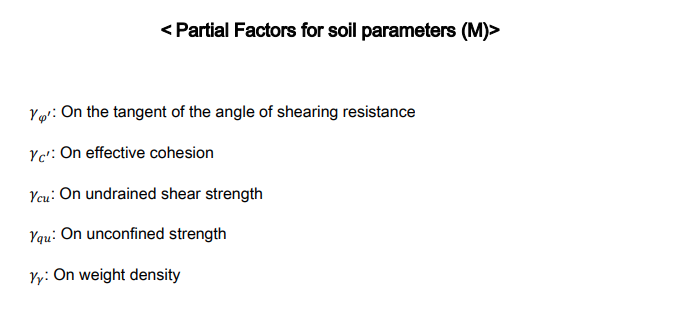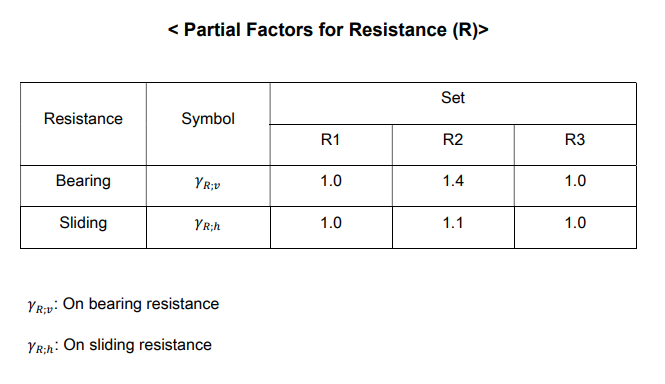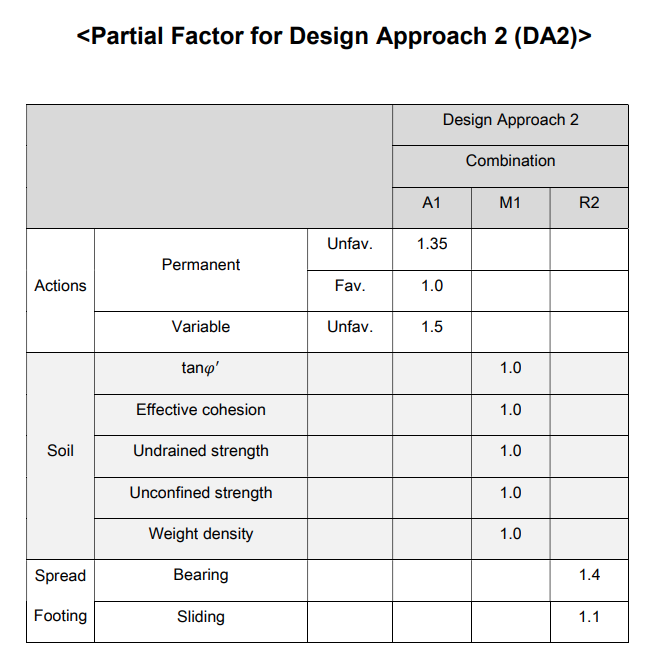• GeoXD
• GTS NX
Overview Geometry Modeler Mesh Generator Solver System Post-Processing
• Events
• Resources
Blog MIDAS Expert Network Technical Support
• Get Started
GeoXD GTS NX
• Talk to us01- Design Approaches

There are three design approach to control the strength of soil, and applied load (surcharge).

Soil strength such as cohesion, friction angle and dilatancy angle will be reduced using the partial factor. Load (surcharge) will be increased with partial factor. Below

There are descriptions about design approaches as below,

Design Approach 1 (DA1)

There are two combinations from Design Approach 1.

Combination 1: A1 + M1 + R1

Combination 2: A2 + M2 + R1

Design Approach 2 (DA2)

Combination: A1 + M1 + R2

Design Approach 3 (DA3)

Combination: (A1 or A2) + M2 + R3

Combinations are denoted by A (for actions or effects of actions), M (for soil parameters) and R (for resistances). They are selected according to the used Design Approaches.

## 02- Partial Factors

#### 1) Partial Factors on action (A)

For the verification of structural (STR) and geotechnical (GEO) limit states set A1 or set A2 of the following partial factors on action or the effects of actions shall be applied. The recommended values for buildings in EN 1990 for the two sets A1 and A2 are given in table below,

####### 2) Partial Factors for soil parameters (M)

For the verification of structural (STR) and geotechnical (GEO) limit state set M1 or set M2 of the following partial factors on soil parameters shall be applied.The recommended values for the two sets M1 and M2 are given in table below,

####3) Partial Factors for Resistance (R)

For spread foundations and verifications of structural (STR) and geotechnical (GEO) limit states, set R1, R2 and R3 of the following partial factors on resistance shall be applied. The recommended values for the three sets R1, R2 and R3 are given in table below,The recommended values for partial factor can be summarized in table below,1- What is Eurocode?#### Written by MIDAS GEO TEAM

Want Engineering tips